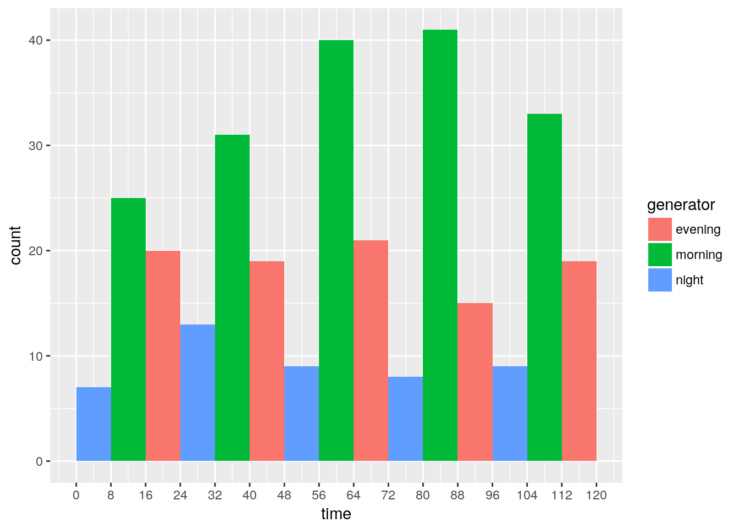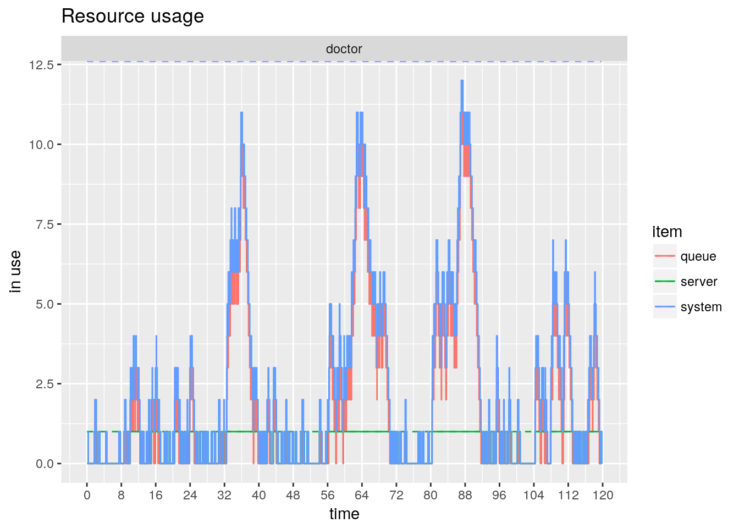# simmer 3.6.1

A new patch release of simmer, the Discrete-Event Simulator for R, is on CRAN. Three months have passed since the last release. The last year was a period of intense development (one release per month). Now, the package has reached some level of maturity, so we intend to extend the release cycle.

In this maintenance release, the replacement operators for trajectories (`[<-`, `[[<-`) now work as expected. Also, we have removed previously deprecated plotting capabilities, which are covered and extended by the simmer.plot package.

Last but not least, we have extended the `from_to()` convenience function with a parameter `every`, which enables the generation of arrivals in cycles. For instance, let us suppose we want to simulate different patient arrival rates in the morning, evening and night:

``````library(simmer)
library(simmer.plot)``````
``## Loading required package: ggplot2``
``````set.seed(1234)

# units are hours
# visit time between 10 and 20 minutes
patient <- trajectory() %>%
seize("doctor", 1) %>%
timeout(function() runif(1, 10/60, 20/60)) %>%
release("doctor", 1)

morning <- from_to(start_time = 8,
stop_time = 16,
dist = function() rexp(1, 60/15),
arrive = FALSE,
every = 24)

evening <- from_to(start_time = 16,
stop_time = 24,
dist = function() rexp(1, 60/30),
arrive = FALSE,
every = 24)

night   <- from_to(start_time = 0,
stop_time = 8,
dist = function() rexp(1, 60/60),
arrive = FALSE,
every = 24)

env <- simmer() %>%
run(24 * 5) # simulate 5 days

breaks <- c(0, cumsum(rep(8, 3 * 5)))

env %>%
get_mon_arrivals() %>%
dplyr::mutate(generator = factor(gsub("\d", "", name))) %>%
ggplot(aes(start_time, fill=generator)) + xlab("time") +
stat_bin(breaks = breaks) +
scale_x_continuous(breaks = breaks)````````````plot(env, what="resources", metric="usage", "doctor", steps=TRUE) +
scale_x_continuous(breaks = breaks)``````### Minor changes and fixes:

• Recycle logical indexes when subsetting (2526e75).
• Implement replacement operators, `[<-` and `[[<-` (#88).
• Provide `rep()` S3 method for trajectories (7fa515e).
• Remove plotting functions (bb9656b), deprecated since v3.6.0. The new `simmer.plot` package (on CRAN) already covers these features among others.
• Don’t evaluate vignette chunks if `Suggests` are not installed (e40e5b6).
• Rewrite DESCRIPTION (3f26516).
• Add an `every` parameter to the `from_to()` convenience function (9d68887).